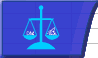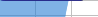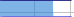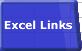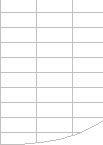# Excel VBA User-Defined FunctionsSmart Recalculation Evaluation Circumstances Calculation Process Dependency Trees Controlling Calculation "CALCULATE" in Status Bar Calculation Methods Calculating from VBA Volatile Functions & Actions User-Defined Functions Repetitive Calculation Version Timing ComparisonAction Ignored Not Calculating Error Handling, #value, calculated twice Uncalculated cells, False dependencies Performance Function Wizard

## User-Defined Functions

User Defined Functions can provide great power and convenience and appear very simple to write. But there are some problem areas that may need special attention in your UDF coding:

• The UDF code must be in a General Module, not a Sheet Module.
• Action ignored: UDF "does nothing"
• Not recalculated when needed or always recalculating.
• Unexpectedly returns #Value or other error.
• Calculates more than once in a recalculation, the Function Wizard or when entered.
• Slow to calculate.

### UDF action being ignored.

Excel will not allow a UDF written in VBA to alter anything except the value of the cell in which it is entered.
You cannot make a VBA UDF which directly:

• Alters the value or formula or properties of another cell.
• Alters the formatting of the cell in which it is entered.
• Alters the environment of Excel. This includes the cursor.
• Uses FIND, SpecialCells, CurrentRegion, CurrentArray, GOTO, SELECT, PRECEDENTS etc : although you can use Range.End.
• Note you can use FIND in Excel 2002/2003.

### UDF not recalculating or always recalculating or calculating in an unexpected sequence:

#### Dependency Sequence Problems

Excel depends on analysis of the input arguments of a Function to determine when a Function needs to be evaluated by a recalculation. What this means is that the function will only be flagged as needing evaluation when one or more of the input arguments change (unless the function is volatile).
If your UDF gets input values from any cells that are not in its argument list then it may not be recalculated, and give the wrong answer. Mostly you can bypass this problem by doing a Full Calculation (Ctrl-Alt-F9), rather than a recalculation (F9), or by making your UDF volatile, but I strongly recommend that you put all the input cells in the argument list.
During a recalculation if Excel does evaluate the UDF it determines which cell references are actually being used inside the function to affect the function result, and if those cells have not yet been finally calculated it will reschedule the Function for later calculation. This is required to make the UDF be finally calculated in the correct dependency sequence.

Function mySum1(SheetName as string) as Variant
mySum1=Worksheets(Sheetname).Range("A1")+worksheets("DATA").Range("A2")
End Function

This function will not automatically recalculate if Data!A2 changes: it will only automatically recalculate when Sheetname changes.

Note that putting all the references in the argument list does not control the initial sequence which Excel uses to calculate the UDF, and it may well be calculated more than once per recalculation cycle.
See UDFs evaluated more than once per workbook calculation.

#### False Dependencies

If you put a false dependency in the argument list (a reference which is not actually used inside the function) Excel will execute the function when the dependency changes, but not neccessarily in dependency sequence.
To ensure that the code in a Function is executed in dependency sequence you need to use ISEMPTY to check the input argument(s). See Detecting Uncalculated Cells below.

#### User-Defined Volatile Functions

 Making UDFs Volatile is NOT a good substitute for including ALL its inputs in the argument list. If you develop a User Defined Function (UDF) you may need to make it Volatile (Application.Volatile(True)) to make it recalculate BUT: Application.Volatile makes your function ALWAYS recalculate each time Excel calculates, which can slow down calculation. Application.Volatile does not directly affect Calculation Sequence. If your UDF refers to cells that are not included in the functions argument list and (if the function is calculated) those cells have not yet been calculated, then Excel will reschedule the UDF to be recalculated again later. Note that uncalculated cells do not stop your UDF from calculating, they just make it calculate more than once. This will mostly give you the correct answer. To make your function volatile put Application.Volatile(True) in your function before any other executable statements. Function mySum2(SheetName as string) as Variant   Application.Volatile(True)   mySum2=Worksheets(Sheetname).Range("A1")+worksheets("DATA").Range("A2") end function MySum will now give you the answer you expect. I recommend avoiding the use of Application.Volatile if at all possible: put ALL your references in the argument list Function mySum3(theFirstCell as range,theSecondCell as range) as Variant   mySum3=thefirstCell.Value+theSecondCell.value End Function

#### Modifying UDFs and Sheet.calculate

Whenever you modify the code in a UDF Excel flags all instances of the UDF in all open workbooks as being uncalculated. If you then do a sheet.calculate or shift/F9 Excel will not only calculate the currently selected worksheet(s), but will also evaluate all the instances of the UDF that are not on the currently selected worksheets.

### Unexpectedly returning #Value, the wrong answer, or being recalculated more than once.

#### UDFs may be evaluated more than once per workbook calculation

 Writing efficient and robust UDFs is not always simple. Various conditions may cause Excel to evaluate your UDF more than once during a recalculation. This means that your UDF should: Explicitly initialize all variables used in the UDF. Error-handle input from uncalculated cells (check for ISEMPTY, unexpected zeros, blanks, missing properties etc) Avoid doing intensive calculations until all input cells have been fully calculated Cache input values and output values to avoid time-intensive calculations when the input values have not changed.

#### Untrapped Errors

You should make sure that you have an On Error handler in your UDF to handle both real errors and errors that are caused by out of sequence execution. Un-trapped errors can halt the calculation process before it is complete.

Function mySum4(theFirstCell as range,theSecondCell as range) as Variant
On error goto FuncFail:
mySum4=thefirstCell.Value+theSecondCell.value
Exit Function
FuncFail:
mySum4=CvErr(xlErrValue)
End Function

#### Using UDFs in Conditional Formats

UDFs referenced in conditional formats get executed each time the screen is refreshed (even if Application.screenupdating=false), and breakpoints in these UDFs will be ignored. Make sure you have an On Error handler in all UDFs referenced in conditional formats, since unhandled errors during a UDF execution caused by a screen refresh may cause VBA to silently stop running without any error message being issued.

Because UDFs referenced by conditional formatting will be executed more frequently than you would expect it is not a good idea to reference slow-running UDFs from conditional formats.

#### Unhandled UDF Errors, and debugging your UDF's

If you don't have an On Error handler in your UDF you may need to be aware of the differences in the way that different Excel versions react.

Excel97

• Excel 97 (SR2 and previous) will interrupt calculation and return #Value for UDF's if:
• Calculation is called from VBA using Sheet.Calculate, Application.Calculate, or SendKeys "%^{F9}", True and the UDF contains an unhandled error
• if Application.Interactive=False Excel will hang, and you will need to shut it down.
• Range.Calculate with Excel 97 will automatically go into debug mode highlighting the error.

If you start the calculation process using Shift-F9 or F9 this problem does not occur: all cells are calculated and debug mode is not entered.

For the Microsoft view on the Excel 97 error handling problem see MSKB 244466

Excel2000

• Range.Calculate goes into debug mode when the UDF has an unhandled error.
• Sheet.Calculate, Application.Calculate and Application.CalculateFull: does not usually interrupt calculation or enter Debug mode.

Excel 2002/2003

• Range.calculate, Sheet.Calculate, Application.Calculate and Application.CalculateFull: does not usually interrupt calculation or enter Debug mode.

#### Detecting Uncalculated Cells, Controlling calculation sequence using false dependencies.

Using the Visual Basic ISEMPTY function on a UDF Range argument will return TRUE if either the input cell is not yet calculated or it contains nothing.

To distinguish between uncalculated cells and cells without any data check that the length of the formula is greater than 0 (suggested by David Cuin) or use Cell.HasFormula:

=ISEMPTY(Cell.Value) AND Len(Cell.formula)>0 or =ISEMPTY(Cell.Value) and Cell.HasFormula

These expressions will only return true if the cell is both uncalculated and contains a formula.

To check whether any of the cells in a range or function input parameter are uncalculated use the following function:

Public Function IsCalced(theParameter As Variant) As Boolean
'
' Charles Williams 9/Jan/2009
'
' Return False if the parameter refers to as-yet uncalculated cells
'
Dim vHasFormula As Variant

IsCalced = True
On Error GoTo Fail
If TypeOf theParameter Is Excel.Range Then
vHasFormula = theParameter.HasFormula
'
' HasFormula can be True, False or Null:
' Null if the range contains a mix of Formulas and data
'
If IsNull(vHasFormula) Then vHasFormula = True
If vHasFormula Then
'
' CountA returns 0 if any of the cells are not yet calculated
'
If Application.WorksheetFunction.CountA(theParameter) = 0 Then IsCalced = False
End If
ElseIf VarType(theParameter) = vbEmpty Then
'
' a calculated parameter is Empty if it references uncalculated cells
'
IsCalced = False
End If
Exit Function
Fail:
IsCalced = False
End Function

I recommend that ALL UDFs should include both an On Error handler and where possible check for empty cells. UDFs written using the C API can check for xlCoerce returning xlretUncalced.

In this example the Function will not return a recalculated answer until both the input arguments have been recalculated and are not empty.

Function mySum5(theFirstCell as Variant,theSecondCell as variant) as Variant
On error goto FuncFail:
If not IsCalced(theFirstCell) or not IsCalced(theSecondCell) then
Exit Function
else
mySum5=thefirstCell.Value+theSecondCell.value
endif
Exit Function
FuncFail:
mySum5=CvErr(xlErrValue)
End Function

If you have a function that you want to calculate in dependency sequence for an argument that does not affect the result of the function (false dependency), you also need to check the input arguments with ISEMPTY.
In this example the second argument is a false dependency because it does not affect the result of the function.

Function mySumTimes2(theFirstCell as range,theSecondCell as range) as Variant
On error goto FuncFail:
If IsEmpty(theFirstCell) or IsEmpty(theSecondCell) then
Exit Function
else
mySumTimes2=thefirstCell.Value*2
endif
Exit Function
FuncFail:
mySumTimes2=CvErr(xlErrValue)
End Function

#### If you are using Excel 97 make sure you have installed the SR1 and SR2 updates.

There are several problems with UDFs in Excel97 which are fixed by these two service releases, which are available from:

#### UDF error with multi-area range argument.

Howar Kaikow http://www.standards.com has discovered a bug in Excel's processing of UDFs using non-contiguous multi-area ranges as input arguments. See AreasBugBypass2.zip for a download containing two workbooks that illustrates the problem and a way of bypassing it..

The problem occurs when:

• A UDF has a multi-area range as one of its input arguments.
• And the multi-area range refers to the worksheet that contains the formula with the UDF.
• And a different sheet is the activesheet when the UDF is calculated.

In these circumstances Excel/VBA incorrectly treats the multi-area range as referring to the active sheet. This means that the UDF may give incorrect answers without warning.

I recommend that you do not attempt to program a UDF to handle multi-area ranges as arguments: use multiple (optional if there are a varying number) range arguments instead.

If required you can bypass the problem as demonstrated in in the download, or by ensuring that there are no instances of the UDF where the multi-area range refers to the sheet with the UDF formula.

The bug exists in Excel 97, Excel 2000, Excel 2002 and partially in Excel 2003. It has been fixed in Excel 2007.

#### Referencing cell formatting properties

If your UDF references cell properties other than .value or .formula (ie .Bold) there are some occasions when these properties may be undefined when your UDF is evaluated. One such occasion is renaming a worksheet in automatic mode. If this happens you may need to explicitly recalculate your function.

### UDF Performance

For optimum performance UDFs should be coded in C and use the C API.
Usually Excel's built-in functions are faster than the equivalent function written in VBA, unless the VBA function uses a better algorithm.

Make sure you use an IsEmpty routine at the top of your UDF to check for uncalculated cells so that you can avoid unneccessary calculations of your UDF. IsEmpty returns True if the variable being checked has not been initialised or has been set to empty. When used on UDF input range arguments IsEmpty returns True if the range either contains nothing or has not yet been calculated. Combining an ISEMPTY test with a test that the formula length is >0 will detect only uncalculated cells but not cells that are empty because they contain nothing.

Also since executing UDF's is usually slower than other Excel calculations, try to put the reference to your UDF in a place in your formulae where it will be calculated as late as possible (towards the end of the formulae and inside the brackets).

Consider if it makes sense only to abandon calculation not only if ALL the input arguments are uncalculated/empty but also if ANY of them are uncalculated/empty.

#### Automatic and Function key Calculation slower than VBA calculation

UDFs calculate significantly slower when the calculation is started automatically by Excel or by pressing F9 than when the calculation is started by a vba calculation statement like Application.calculate.
The slowdown is significantly larger if the VBE is open and not minimised.
The slowdown is an overhead for each UDF that is recalculated, so its roughly proportional to the number of UDFs.

These timings are for 16000 very simple UDFs, using Excel 2002 on an AMD 1200MHZ with Windows XP:

 Autocalc with VBE open and maximised 91 seconds Autocalc with VBE open and minimised 38 seconds Autocalc with VBE closed 2 seconds Application.Calculatefull with VBE open and maximised 0.302 seconds Application.Calculatefull with VBE closed 0.293 seconds

So if you are using a lot of UDFs it really pays to be in manual calculation mode and have a calculate button that uses VBA to initiate an Excel calculation (Application.Calculate or Application.Calculatefull).

UDFs in Automation addins created using VB6 do not use the VBE, so do not suffer from the VBE overhead as above, but otherwise give very similar performance to VBA. In theory compiled VB6 with array bounds checking disabled should be faster for intensive arithmetic calculations on arrays, but I have not yet managed to detect any speed improvement.

#### Array Functions

UDF's can be written as multicell array formulae that can be entered using Ctrl-Shift-Enter. The results of the array calculation are returned to the cells by assigning an array to the function.
Note that Excel behaves unexpectedly when a multi-cell UDF is entered or modified and depends on volatile formulae: the UDF is evaluated once for each cell it occupies. This does not happen when the UDF is recalculated, only when it is entered or changed.

#### Transferring information from Excel Ranges to the UDF.

You should also try to minimise the performance overhead of transferring information from Excel to VBA and back to Excel:
If you are going to process each cell in the input range(s) then they should usually be read into a variant variable containing an array, which is subsequently read from for the calculations. This avoids reading information from Excel cell by cell, which is slow, and ensures that you only read each cell once. Note that this example assumes that each range is a single-area contiguous range.

It is faster (15-20%) to use the Range.Value2 property rather than the (default) Range.Value property. The Range.Value property attempts to convert cells formatted as Dates to a variant containg a VBA date type, and cells formatted as currency to a variant containing a VBA Currency type. Range.value2 attempts to convert date and Currency formatted cells into Variants containing Doubles.

Function mySum6(theFirstRange As Range, theSecondRange As Range) As Variant

Dim dblMySum As Double
Dim varRange1 As Variant
Dim varRange2 As Variant
Dim j As Long
Dim k As Long

On Error GoTo FuncFail:
'
' initialise output
'
dblMySum = 0#

'
' check for non-empty cells
'

If not IsCalced(theFirstRange) or not IsCalced(theSecondRange) then exit Function
'
' get ranges into variant variables holding array
'
varRange1 = theFirstRange.Value2
varRange2 = theSecondRange.Value2

blEmptyCells = True
For j = 1 To UBound(varRange1, 1)
If Not blEmptyCells Then Exit For
For k = 1 To UBound(varRange1, 2)
If Not IsEmpty(varRange1(j, k)) Then
blEmptyCells = False
Exit For
End If
Next k
Next j
If blEmptyCells Then
For j = 1 To UBound(varRange2, 1)
If Not blEmptyCells Then Exit For
For k = 1 To UBound(varRange2, 2)
If Not IsEmpty(varRange2(j, k)) Then
blEmptyCells = False
Exit For
End If
Next k
Next j
End If
'
' exit function if ALL input cells are empty
'
If blEmptyCells Then
Exit Function
Else
'
' add cells to double (error traps text cells)
'
For j = 1 To UBound(varRange1, 1)
For k = 1 To UBound(varRange1, 2)
dblMySum = dblMySum + varRange1(j, k)
Next k
Next j
For j = 1 To UBound(varRange2, 1)
For k = 1 To UBound(varRange2, 2)
dblMySum = dblMySum + varRange2(j, k)
Next k
Next j
End If
'
' assign value to function
'
mySum6 = dblMySum
Exit Function
FuncFail:
mySum6 = CVErr(xlErrValue)
End Function

#### Using Excel functions inside your UDF.

If you are going to process the input ranges using Excel functions called from VBA, then keep the input ranges as Range object variables, so that the function does not have to transfer all the cell information to VBA : you are then just manipulating the object variable pointers.

Note that functions which reference ranges such as VLOOKUP and INDEX can return Empty without raising an error if the range being referenced contains uncalculated cells.
Since assigning Empty to a Long results in zero you can get unexpected results if you do not check for this condition.

Note that using Application.WorksheetFunction.Match is generally about 20% faster than using Application.Match (similar results expected for other functions). The other difference is that Application.WorksheetFunction raises an error if, for instance, no match is found, but Application.Match returns an error value without raising an error.

This example extends the isempty check to disjoint ranges containing multiple areas, and will only check cells that are within the used range (efficient handling of ranges specified as entire columns or rows).

Note that because there is a bug in the way Excel handles ranges containing multiple areas I do not recommend that UDF's are programmed to handle multiple areas.

Function mySum7(theFirstRange As Range, theSecondRange As Range) As Variant
Dim blEmptyCells As Boolean
Dim rngUsedCellsInRange As Range
Dim oCell As Range
Dim j As Long
On Error GoTo FuncFail:
'
' check for non-empty cell
'
blEmptyCells = True
Set rngUsedCellsInRange = Intersect(theFirstRange, theFirstRange.Parent.UsedRange)
If Not rngUsedCellsInRange Is Nothing Then
For Each oCell In rngUsedCellsInRange
If Not IsEmpty(oCell) Then
blEmptyCells = False
Exit For
End If
Next oCell
End If
If blEmptyCells Then
Set rngUsedCellsInRange = Intersect(theSecondRange, theSecondRange.Parent.UsedRange)
If Not rngUsedCellsInRange Is Nothing Then
For Each oCell In rngUsedCellsInRange
If Not IsEmpty(oCell) Then
blEmptyCells = False
Exit For
End If
Next oCell
End If
End If
Set oCell = Nothing
Set rngUsedCellsInRange = Nothing
'
' exit function if all input cells are empty
'
If blEmptyCells Then
Exit Function
Else
mySum7 = Application.Sum(theFirstRange, theSecondRange)
End If
Exit Function
FuncFail:
Set oCell = Nothing
Set rngUsedCellsInRange = Nothing
mySum7 = CVErr(xlErrValue)
End Function

#### UDFs with a variable number of input arguments.

This example shows how to handle a variable number of arguments.

Function mySum8(ParamArray varArgs() As Variant) As Variant

Dim varArg As Variant
Dim rngCell As Range
Dim dblMySum As Double
Dim blEmptyCells As Boolean
Dim j As Long
Dim k As Long

On Error GoTo FuncFail:
'
' initialise output
'
dblMySum = 0#
'
' check for non-empty cell
'
blEmptyCells = True
For Each varArg In varArgs
If Not blEmptyCells Then Exit For
If Not IsMissing(varArg) Then
'
' if not a range skip the check
'
If TypeName(varArg) = "Range" Then
For Each rngCell In varArg
If Not blEmptyCells Then Exit For
If Not IsEmpty(rngCell) Then
blEmptyCells = False
Exit For
End If
Next rngCell
Else
If Not IsEmpty(varArg) Then blEmptyCells = False
End If
End If
Next varArg
'
' exit function if all input cells are empty
'
If blEmptyCells Then
Exit Function
Else
'
' add cells to double (error traps text cells)
'
For Each varArg In varArgs
If Not IsMissing(varArg) Then
If TypeName(varArg) = "Range" Then
For Each rngCell In varArg
dblMySum = dblMySum + rngCell
Next rngCell
Else
dblMySum = dblMySum + varArg
End If
End If
Next varArg
End If
'
' assign value to function
'
mySum8 = dblMySum
Exit Function
FuncFail:
mySum8 = CVErr(xlErrValue)
End Function

### Function Wizard

Your end-users can use the Function Wizard to enter your UDFs (usually from the User Defined Category) into a worksheet.

If your UDF takes a long time to execute you will soon discover that the Function wizard executes your UDF several times.
There are two methods you can use to avoid this:

• Declare the function as Private: the UDF will still work but will not appear in the list of functions shown by the function wizard (but you can still use the Function Wizard to modify an existing entry).
• Add code to your UDF to determine if it has been called from the function Wizard.

Axel König has suggested adding the following code:

If (Not Application.CommandBars("Standard").Controls(1).Enabled) Then Exit Function

This code depends on the fact that when using the function wizard most icons in the toolbars are disabled. I have tested Axel's solution in Excel 97, Excel 2000, Excel 2002 and Excel 2003, and it works well.

A solution is also possible by using the Windows API to check if the Function Wizard window is showing and has the same process ID as the current Excel process.© 2001-2020 Decision Models Legal Disclaimer Privacy Statement